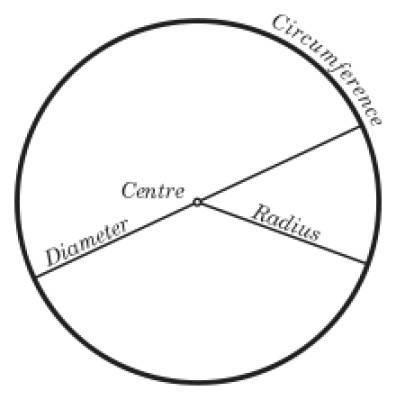List of public pages created with Protopage

# Home

## Plain sticky notes

### Rules

The rules of adding algabra are: A plus and a plus = a posivtive number A minus and a minus = a positive number A plus and minus = a minus when means its negative number

### Algabra words

Simplify- get rid of the brackets Solve- Find the value Equation- contains maths signs Evaluate- Simplify and find the value

### BIDMAS- Order of calculation when solving equations

Brackets- do the brackets first Indices-Numbers out side the brackets Division Multiplication Adding Subtraction

### Sticky note

Mymaths is a useful website to practiceyour maths

# Practice

## Plain sticky notes

### Have a go.....

Solve the following equation. -5 + - 6 Solve the following equation. -5 + - 8 + - 6 Solve the following equation. -7 + - 5 Solve the following equation. 2x + 2 = x + 4 Solve the following equation. 5x - 2 = 12 - 2x Solve the following equation. 2(5x + 1) = 3(3x + 7) Solve the following equation. 3(2x - 5) = 3(x +1)

# Notes

## Plain sticky notes

### Sticky note

Click this text to change it...

# Circles

## Plain sticky notes

### circumference

To work out the circumfrerence you do pie(3.142)xdiameter for example if the diameter was 10 you would do 3.142 x 10=31.42

### Area of a circle

pie x radius = area for .example 100 = the diameter half that to get the radius 50 then 3.142 x 50= 157.1

## Photos

### circle